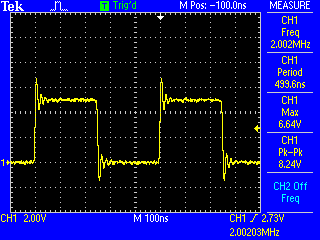# pwm arduino

hi. im a very beginner to arduino. i would like to ask about generating pwm using arduino, i have this code for ATmel as below that generate 8.2us of period or 121kHz frequency.. i've tried to use the codes n test it using oscilloscope in arduino, and it wont do..how can i modified the codes for me to be able to obtain same signal frequency using arduino 0023.. please help me. thank u so much..

#include <avr/io.h>
#define F_CPU 16000000UL //set 16mhz crystal

int main (void)
{
DDRD |=0xFF;

//initialization for timer1
TCCR1A |= 1<<WGM11 | 1<<COM1A1 | 0<<COM1A0; // COM1A1=1,COM1A0=0,FOR NON INVERTED MODE...1:1 FOR INVERTED MODE
TCCR1B |= 1<<WGM12 | 1<<WGM13 | 1<<CS10 | 1<<ICNC1; //sebelum ni CS10 =1;ICNC1 =1 noise canceller
ICR1 = 7; //counter value utk 16MHZ

OCR1A = 3;
}

DDRD |=0xFF;

Since this is in the main loop, it does nothing, repeatedly ORing in all 1's on top of 1's.
Perhaps you want XOR, which toggles.

Also, why is init code in the main loop, rather than init routine.

Since this is in the main loop, it does nothing, repeatedly

Read it againhaneys:
i have this code for ATmel as below that generate 8.2us of period or 121kHz frequency

How do you calculate that? You are counting up to 8 with a duty cycle of 4. So you have a 50% duty cycle with a period of 500 nS, giving a frequency of 2 MHz. It outputs on pin 9 so you need:

``````   DDRB |= 0xFF;
``````

Personally I prefer:

``````pinMode (9, OUTPUT);
``````

@OP, posting the same question in different parts of the forum is not a good way to make a good first impression; it simply wastes everyones time.
I've deleted the duplicate.

Oh? I didn't notice that. Just as well, eh?

Anyway, proof of what happens on pin 9:@awol, sorry my bad, its my first time joining forum..i c.. then how can i write it using arduino, i tried as below, its not working n no signal shows up. correct me please.. and actually what should i put in void loop().. many2 thankz!! :.

void setup() {
pinMode(9, OUTPUT);

TCCR1A |= _BV(WGM11) | _BV(COM1A1);
TCCR1B |= _BV(WGM12) | _BV(WGM13) | _BV(CS10) | _BV(ICNC1);

ICR1= 7;

OCR1A = 3;}

void loop(){
}

Try:

``````void setup() {
pinMode(9, OUTPUT);

TCCR1A = 0;
TCCR1B = 0;

TCCR1A |= _BV(WGM11) | _BV(COM1A1);
TCCR1B |= _BV(WGM12) | _BV(WGM13) | _BV(CS10) | _BV(ICNC1);

ICR1= 7;

OCR1A = 3;
}

void loop(){
}
``````

The Arduino IDE initializes Timer 1 to do stuff. You need to stop all that before you start "oring" in new values.

Or try this:

``````void setup()
{
pinMode(9, OUTPUT);

TCCR1A = _BV(WGM11) | _BV(COM1A1);
TCCR1B = _BV(WGM12) | _BV(WGM13) | _BV(CS10) | _BV(ICNC1);
ICR1= 7;
OCR1A = 3;
}

void loop() {}
``````

If you are going to "|=" things you need to be pretty certain about what the existing values are.

if comparing these two codes, do they obtain similar signal? because as the first code there is 0<<COM1A0, since COM1A0 = 0, i just exclude it in the second code..i have to go to lab to use oscilloscope,but lab close after 5.if u dont mind, may i know is there any software u used to get the previous image result one..

1st code:

``````#include <avr/io.h>
#define F_CPU 16000000UL //set 16mhz crystal

int main (void)
{
DDRD |=0xFF;

//initialization for timer1
TCCR1A |= 1<<WGM11 | 1<<COM1A1 | 0<<COM1A0; // COM1A1=1,COM1A0=0,FOR NON INVERTED MODE...1:1 FOR INVERTED MODE
TCCR1B |= 1<<WGM12 | 1<<WGM13 | 1<<CS10 | 1<<ICNC1; //sebelum ni CS10 =1;ICNC1 =1 noise canceller
ICR1 = 7; //counter value utk 16MHZ

OCR1A = 3;
}
``````

2nd code:

``````void setup() {
pinMode(9, OUTPUT);

TCCR1A = 0;
TCCR1B = 0;

TCCR1A |= _BV(WGM11) | _BV(COM1A1);
TCCR1B |= _BV(WGM12) | _BV(WGM13) | _BV(CS10) | _BV(ICNC1);

ICR1= 7;

OCR1A = 3;
}

void loop(){
}
``````

btw, tq tq tq..

haneys:
the first code there is 0<<COM1A0, since COM1A0 = 0, i just exclude it in the second code..

That's right. No difference.

ok. thankz a lot..Also equivalent and more concise is:

``````void setup() {
pinMode(9, OUTPUT);

TCCR1A = _BV(WGM11) | _BV(COM1A1);
TCCR1B = _BV(WGM12) | _BV(WGM13) | _BV(CS10) | _BV(ICNC1);

ICR1= 7;
OCR1A = 3;
}
``````

tq for helping everyone..